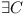'. '

# LibraryReExportIsNPComplete

This page describes a way to convert any wikipedia::3SAT problem to a solution of finding the right configuration from conflicting libraries in a system that can re-export APIs. Thus proving that the later problem is wikipedia::NP-complete.

## wikipedia::3SAT

The problem of satisfying a logic formula remains NP-complete even if all expressions are written in wikipedia::conjunctive normal form with 3 variables per clause (3-CNF), yielding the 3SAT problem. This means the expression has the form:$(x_{11} \vee x_{12} \vee x_{13}) \wedge$$(x_{21} \vee x_{22} \vee x_{23}) \wedge$$(x_{31} \vee x_{32} \vee x_{33}) \wedge$
...$(x_{n1} \vee x_{n2} \vee x_{n3})$

where each xab is a variable vi or a negation of a variable$\neg v_i$. Each variable vi can appear multiple times in the expression.

## Module Dependencies Problem

Let A,B,C,... denote an API.

Let A1,A1.1,A1.7,A1.11 denote compatible versions of API A.

Let A1,A2.0,A3.1 denote incompatible versions of API A.

Let Ax.y > Bu.v denote the fact that version x.y of API A depends on version u.v of API B.

Let$A_{x.y} \gg B_{u.v}$ denote the fact that version x.y of API A depends on version u.v of API B and that it re-exports B's elements.

Let Repository R = (M,D) be any set of modules with their various versions and their dependencies on other modules with or without re-export.

Let C be a Configuration in a repository R = (M,D), if$C \subseteq M$, where following is satisfied:$\forall A_{x.y} \in C, \forall A_{x.y} \gg B_{u.v} \in D \Rightarrow \exists w >= v \wedge B_{u.w} \in C$ - each re-exported dependency is satisfied with some compatible version$\forall A_{x.y} \in C, \forall A_{x.y} > B_{u.v} \in D \Rightarrow \exists w >= v B_{u.w} \in C$ - each dependency is satisfied with some compatible version
Let there be two chains of re-exported dependencies$A_{p.q} \gg ... \gg B_{x.y}$ and$A_{p.q} \gg ... \gg B_{u.v}$ then$x = u \wedge y = v$ - this guarantees that each class has just one, exact meaning for each importer

#### Module Dependency Problem

Let there be a repository R = (M,D) and a module$A \in M$. Does there exist a configuration C in the repository R, such that the module$A \in C$, e.g. the module can be enabled?

## Converstion of wikipedia::3SAT to Module Dependencies Problem

Let there be wikipedia::3SAT formula with with variables v1,...,vm as defined above.

Let's create a repository of modules R. For each variable vi let's create two modules$M^i_{1.0}$ and$M^i_{2.0}$, which are mutually incompatible and put them into repository R.

For each formula$(x_{i1} \vee x_{i2} \vee x_{i3})$ let's create a module Fi that will have three compatible versions. Each of them will depend on one variable's module. In case the variable is used with negation, it will depend on version 2.0, otherwise on version 1.0. So for the formula$v_a \vee \neg v_b \vee \neg v_c$

we will get:$F^i_{1.1} \gg M^a_{1.0}$$F^i_{1.2} \gg M^b_{2.0}$$F^i_{1.3} \gg M^c_{2.0}$

All these modules and dependencies add into repository R

Now we will create a module T1.0 that depends all formulas:$T_{1.0} \gg F^1_{1.0}$$T_{1.0} \gg F^2_{1.0}$
...$T_{1.0} \gg F^n_{1.0}$

and add this module as well as its dependencies into repository R.

Claim: There$\exists C$ (a configuration) of repository R and$T_{1.0} \in C$$\Longleftrightarrow$ there is a solution to the wikipedia::3SAT formula.

## Proof

"$\Leftarrow$": Let's have an evaluation of each variable to either true or false that evaluates the whole wikipedia::3SAT formula to true. Then$C = \{ T_{1.0} \} \bigcup$$\{ M^i_{1.0} : v_i \} \bigcup \{M^i_{2.0} : \neg v_i \} \bigcup$$\{ F^i_{1.1} : x_{i1} \} \bigcup \{ F^i_{1.2} : \neg x_{i1} \wedge x_{i2} \} \bigcup \{ F^i_{1.3} : \neg x_{i1} \wedge \neg x_{i2} \wedge x_{i3} \}$

It is clear from the definition that each Mi and Fi can be in the C just in one version. Now it is important to ensure that each module is present always at least in one version. This is easy for Mi as its vi needs to be true or false, and that means one of$M^i_{1.0}$ or$M^i_{2.0}$ will be included. Can there be a Fi which is not included? Only if$\neg x_{i1} \wedge \neg x_{i2} \wedge \neg x_{i3}$ but that would mean the whole 3-or would evaluate to false and as a result also the wikipedia::3SAT formula would evaluate to false. This means that dependencies of T1.0 on Fi modules are satisfied. Are also dependencies of every$F^i_{1.q}$ satisfied? From all the three versions, there is just one$F^i_{1.q}$, the one its xiq evaluates to true. However xiq can either be without negation, and as such$F^i_{1.q}$ depends on$M^j_{1.0}$ which is included as vj is true. Or xiq contains negation, and as such$F^i_{1.q}$ depends on$M^j_{2.0}$ which is included as vj is false. qed.